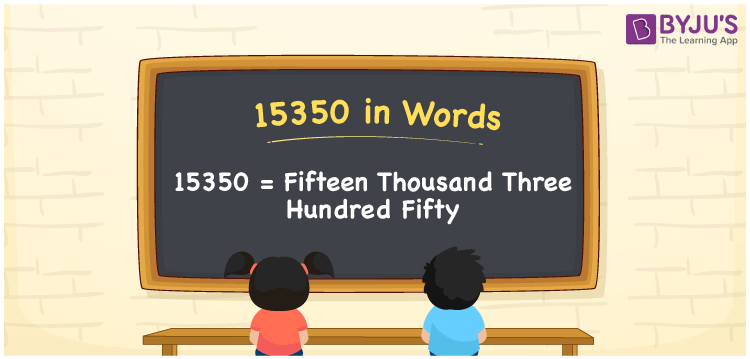# 15350 in Words

15350 in words is written as Fifteen thousand three hundred fifty. In both the International System of Numerals and the Indian System of Numerals, 15350 is written as Fifteen thousand three hundred fifty. The number 15350 is a Cardinal Number as it could describe some quantity. For example, “that smartphone costs 15350 rupees”.

 15350 in words Fifteen thousand three hundred fifty Fifteen thousand three hundred fifty in Number 15350

## 15350 in English Words

15350 in English words is read as “Fifteen thousand three hundred fifty”.## How to Write 15350 in Words?

To write 15350 in words, we shall use the place value chart. In the place value chart, put 1 in the ten thousand, 5 in the thousands, 3 in the hundreds, 5 in the tens and 0 in the ones. Let us make a place value chart to write the number 15350 in words.

 Ten Thousands Thousands Hundreds Tens Ones 1 5 3 5 0

Thus, we can write the expanded form as

1 × Ten Thousand + 5 × Thousand + 3 × Hundred + 5 × Ten + 0 × One

= 1 × 10000 + 5 × 1000 + 3 × 100 + 5 × 10 + 0 × 1

= 10000 + 5000 + 300 +50 + 0

= 15350

= Fifteen thousand three hundred fifty.

15350 is a natural number, the successor of 15349 and the predecessor of 15351.

15350 in words –Fifteen thousand three hundred fifty

• Is 15350 an odd number? – No
• Is 15350 an even number? – Yes
• Is 15350 a perfect square number? – No
• Is 15350 a perfect cube number? – No
• Is 15350 a prime number? – No
• Is 15350 a composite number? – Yes

## Frequently Asked Questions on 15350 in Words

Q1

### How to write 15350 in words?

15350 in words is written as fifteen thousand three hundred fifty.
Q2

### How to write 15350 in the International and Indian System of Numerals?

In both, the system of numerals, 15350 in words, is written as fifteen thousand three hundred fifty.
Q3

### How to write 15350 in a place value chart?

In the place value chart, write 1 in the ten thousand, 5 in the thousands, 3 in the hundreds, 5 in the tens and 0 in the ones, respectively.